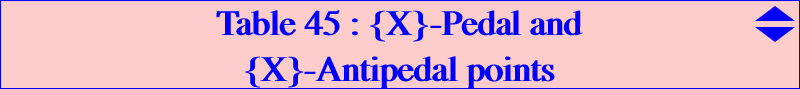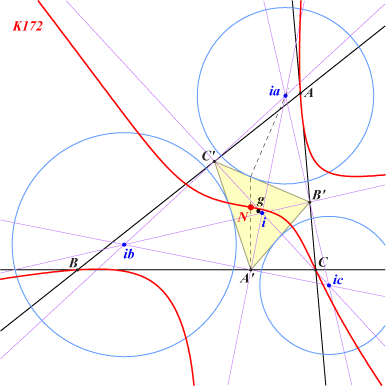Definitions : A point P is an {X}-pedal point if P is a center in {X} with respect to its Pedal triangle. A point P is an {X}-antipedal point if P is a center in {X} with respect to its Antipedal triangle. See Table 28 for cevian equivalents and notations. For example, X(6) is the unique X(2)-pedal point since it is the centroid of its pedal triangle and the in/excenters of ABC are the X(4)-antipedal points since each one is the orthocenter of the triangle formed by the three others. Let P = u : v : w be a point and P* its isogonal conjugate (in ABC). We denote pedP and apdP the pedal and antipedal triangles of P as in Table 6. We first recall some properties we shall use in the sequel. • The product of the areas of pedP and apdP* is the square of the area ∆ of ABC. • The triangles pedP and apdP* are homothetic and the center of the homothety is P' = a^2(b^2 u + SC v)(c^2 u + SB w) : : . • This homothety h maps P to h(P) which is the isogonal conjugate of P* with respect to apdP*. This shows that the technique used by Wilson Stothers in Table 28 can be easily adapted here. If a center X is on a strong curve F, then we can define two loci: pF = { Y : Y is an {X}-Pedal point }, and aF = { Y : Y is an {X}-Antipedal point }. The condition that F is strong ensures that it contains all members of {X}. It also means that F can be defined using squares of sidelengths. Since the squares of the sidelengths of Pedal and Antipedal triangles can be computed easily, we can identify the curves pF and aF. For example, suppose that the pedal triangle of Y = x:y:z has sidelengths with squares P, Q, R, and that the coefficients of F are given in terms of a^2, b^2, c^2. Then we simply replace a^2, b^2, c^2 by P, Q, R and x : y : z by its isogonal conjugate in F(x,y,z) = 0 to get an equation for pF. It follows that Y is an {X}-Pedal point if and only if X* is as an {Y}-Antipedal point. Similarly, to get an equation for aF, we replace a^2, b^2, c^2 by P, Q, R (squares of the sidelengths of apdY) and x : y : z by the coordinates of the reflection of Y about the complement of Y*(or equivalently, the anticomplement of the midpoint of Y-Y*) i.e. by x(c^2y^2 + b^2z^2 + 2SA yz) : : . The following table gives a selection of these curves with a list of centers.pF : Locus of {X}-Pedal points X on curve F aF : Locus of {X}-Antipedal points notes sextic Q039 Brocard axis Kiepert hyperbola quartic Q002 Euler line septic Q030 circular quartic / X(6), X(15), X(16) line GK bicircular septic / X(2), X(13), X(14), X(111) Brocard axis Kiepert hyperbola sextic Q039 note 4 quartic / X(4), X(6), X(15), X(16), X(2067), X(2183) Wallace hyperbola bicircular nonic quintic Q037 Jerabek hyperbola quintic Q003 note 5 bicircular octic / X(1), X(4), X(15), X(16) Stammler hyperbola quintic Q050 Lemoine axis Steiner ellipse isogonal bicircular sextic pK(X6, P on Euler line) pK(X6, P on Euler line) pK(X6, P on Euler line) note 1 K172 = pK(X32, X3) Lucas cubic K007 tricircular nonic note 3 circular quintic Q094 Grebe cubic K102 quartic Q019 note 6 nK0(X6, P on X6-X523) nK0(X6, P on X6-X523) nK0(X6, P on X6-X523) note 2 K018 K018 K018 K024 K024 K024note 1 : any strong center X that lies on a cubic of the Euler pencil (see Table 27) whose pivot is (1-t) X3+t X4 (t real number) has its {X}-Pedal and its {X}-Antipedal points on this same cubic. note 2 : any strong center X that lies on an isogonal nK0 with root (1-t) X6+t X523 (t real number) has also its {X}-Pedal and its {X}-Antipedal points on this same cubic. This is the case of K018 and K024. note 3 : the {X}-pedal points of any X on the Lucas cubic K007 must lie on K172 which is the isogonal transform of K007. When X = X(20), we find that the {X20}-pedal points must also lie on Q002 (since X20 is on the Euler line) and on K004 (see note 1). There are five such points : O and the isogonal conjugates of the CPCC points of Table 11. note 4 : The Kiepert hyperbola (K) and the Wallace hyperbola (W) generate a pencil of homothetic conics written under the form (C) = (1-t) (K) +t (W) and (C) passes through G with tangent the line GK. If X lies on (C), the locus of the {X}-Pedal points is a quartic passing through X(6), X(15), X(16). The complement of (K) is a member of the pencil which corresponds to the quartic Q043. note 5 : The Jerabek hyperbola (J) and the Stammler hyperbola (S) also generate a pencil of homothetic conics written under the form (C) = (1-t) (J) +t (S) and, if X lies on (C), the locus of the {X}-Antipedal points is a circular quintic with focus G having three real asymptotes parallel to those of the Thomson cubic K002. This quintic also contains X(4), X(13), X(14), X(1113), X(1114). When (C) decomposes into the line at infinity and the Brocard axis, the quintic splits into the line at infinity, the circumcircle and (K). note 6 : More generally, if F is invariant under isogonal conjugation then aF is the isogonal transform of pF. Examples Example 1 : the {X5}-Pedal points must lie on the Napoleon cubic K005 and on Q002. Hence their isogonal conjugates must lie on K005 and on Q003. These are the Ix-anticevian points. See Table 23. the {X5}-Antipedal points must lie on K005 and on Q030. Hence their isogonal conjugates must lie on K005 and on Q037. Example 2 : the {X20}-Pedal points must lie on the Darboux cubic K004, on K172 and on Q002. Hence their isogonal conjugates must lie on K004, on K007 and on Q003. These are the CPCC points. See Table 11. the {X20}-Antipedal points must lie on K004 and on Q030. Hence their isogonal conjugates must lie on K004 and on Q037.How to deal with weak centers... The technique above works when we know strong curves F passing through the center X. Recall that, when X is weak, F must also contain the three extraversions Xa, Xb, Xc of X. It is then very easy to find infinitely many strong conics passing through X : it is enough to take a member of the pencil of conics passing through X, Xa, Xb, Xc which contains a strong center. Since this pencil contains three decomposed cubics, it is better to use these when they are easily identified. Example : the {X(8)}-Pedal pointsThe weak Nagel point X(8) lies on the Lucas cubic K007 hence the {X(8)}-pedal points already lie on K172. Since X(8) is the incenter of the antimedial triangle, all the conics that contain X(8) and its extraversions are rectangular hyperbolas and three of them are decomposed into two perpendicular lines. One of them is (Ca), the union of the line X(8)Ga and its perpendicular at Ga, where Ga is the A-vertex of the antimedial triangle. These are actually the bisectors at Ga of the antimedial triangle, with equation : b^2(x + y)^2 = c^2(x + z)^2. The corresponding curves pF are three quartics and, by adding them two by two, we obtain three cubics having seven common points : 6 are usually imaginary (except when the triangle ABC is almost "flat") and the seventh is the point N such that the Nagel point of the pedal triangle A'B'C' of N is N. Its SEARCH number is : 1.797342334832492. On the figure, the points g and i, ia, ib, ic are the centroid and the in/excenters of A'B'C'.Remark : with the {X(10)}-Pedal points, the situation is very similar since we also have three decomposed rectangular hyperbolas (recall that X(10) is the incenter of the medial triangle) but, in this case, all the {X(10)}-Pedal points are collinear on the Brocard axis since X(10) lies on the Kiepert hyperbola.